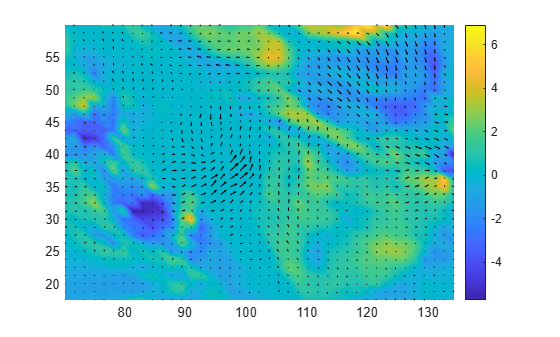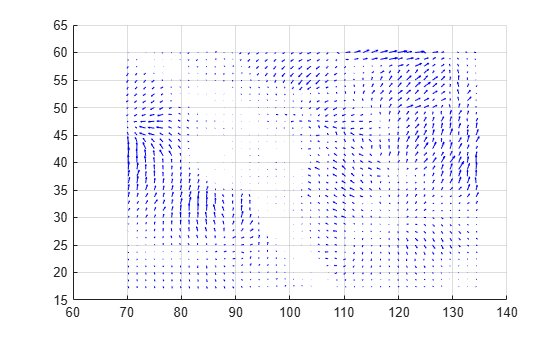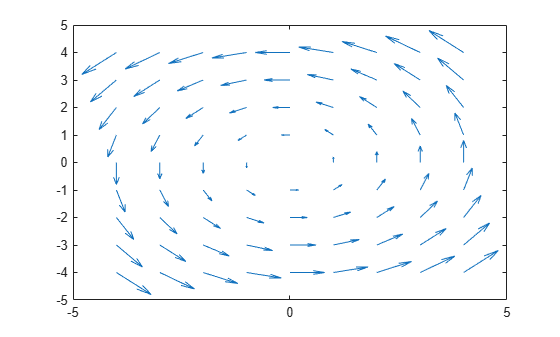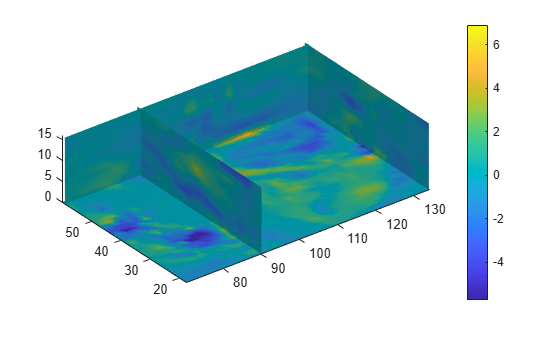# curl

Curl and angular velocity of vector field

## Syntax

``[curlx,curly,curlz,cav] = curl(X,Y,Z,Fx,Fy,Fz)``
``[curlx,curly,curlz,cav] = curl(Fx,Fy,Fz)``
``[curlz,cav] = curl(X,Y,Fx,Fy)``
``[curlz,cav] = curl(Fx,Fy)``
``cav = curl(___)``

## Description

example

````[curlx,curly,curlz,cav] = curl(X,Y,Z,Fx,Fy,Fz)` computes the numerical curl and angular velocity of a 3-D vector field with vector components `Fx`, `Fy`, and `Fz`. The output `curlx`, `curly`, and `curlz` represent the vector components of the curl, and `cav` represents the angular velocity of the curl.The arrays `X`, `Y`, and `Z`, which define the coordinates for the vector components `Fx`, `Fy`, and `Fz`, must be monotonic, but do not need to be uniformly spaced. `X`, `Y`, and `Z` must be 3-D arrays of the same size, which can be produced by `meshgrid`.```
````[curlx,curly,curlz,cav] = curl(Fx,Fy,Fz)` assumes a default grid of sample points. The default grid points `X`, `Y`, and `Z` are determined by the expression `[X,Y,Z] = meshgrid(1:n,1:m,1:p)`, where ```[m,n,p] = size(Fx)```. Use this syntax when you want to conserve memory and are not concerned about the absolute distances between points.```

example

````[curlz,cav] = curl(X,Y,Fx,Fy)` computes the numerical curl and angular velocity of a 2-D vector field with vector components `Fx` and `Fy`. The output `curlz` represents the z-component of the curl, and `cav` represents the angular velocity of the curl.The matrices `X` and `Y`, which define the coordinates for `Fx` and `Fy`, must be monotonic, but do not need to be uniformly spaced. `X` and `Y` must be 2-D matrices of the same size, which can be produced by `meshgrid`.```
````[curlz,cav] = curl(Fx,Fy)` assumes a default grid of sample points. The default grid points `X` and `Y` are determined by the expression ```[X,Y] = meshgrid(1:n,1:m)```, where `[m,n] = size(Fx)`. Use this syntax when you want to conserve memory and are not concerned about the absolute distances between points.```

example

````cav = curl(___)` returns only the angular velocity of the vector field.```

## Examples

collapse all

Load a 3-D vector field data set that represents a wind flow. The data set contains arrays of size 35-by-41-by-15.

`load wind`

Compute the numerical curl and angular velocity of the vector field.

`[curlx,curly,curlz,cav] = curl(x,y,z,u,v,w);`

Show a 2-D slice of the computed curl and angular velocity. Slice the data at `z(:,:,1)`, where the $z$-coordinate is equal to -0.002.

```k = 1; xs = x(:,:,k); ys = y(:,:,k); zs = z(:,:,k); us = u(:,:,k); vs = v(:,:,k); ```

Plot the angular velocity in 2-D coordinates using `pcolor`. Show the $x$- and $y$-components of the vector field using `quiver`.

```pcolor(xs,ys,cav(:,:,k)) shading interp colorbar hold on quiver(xs,ys,us,vs,'k') hold off```Next, plot the components of the 3-D curl at the sliced $xy$-plane.

```quiver3(xs,ys,zs,curlx(:,:,k),curly(:,:,k),curlz(:,:,k),'b') view(0,90)```Specify 2-D coordinates and the vector field.

```[x,y] = meshgrid(-4:4,-4:4); Fx = -y*2; Fy = x*2;```

Plot the vector field components `Fx` and `Fy`.

`quiver(x,y,Fx,Fy)`Find the numerical curl and angular velocity of the 2-D vector field. The values of curl and angular velocity are constant at all input coordinates.

`[curlz,cav] = curl(x,y,Fx,Fy)`
```curlz = 9×9 4 4 4 4 4 4 4 4 4 4 4 4 4 4 4 4 4 4 4 4 4 4 4 4 4 4 4 4 4 4 4 4 4 4 4 4 4 4 4 4 4 4 4 4 4 4 4 4 4 4 4 4 4 4 4 4 4 4 4 4 4 4 4 4 4 4 4 4 4 4 4 4 4 4 4 4 4 4 4 4 4 ```
```cav = 9×9 2 2 2 2 2 2 2 2 2 2 2 2 2 2 2 2 2 2 2 2 2 2 2 2 2 2 2 2 2 2 2 2 2 2 2 2 2 2 2 2 2 2 2 2 2 2 2 2 2 2 2 2 2 2 2 2 2 2 2 2 2 2 2 2 2 2 2 2 2 2 2 2 2 2 2 2 2 2 2 2 2 ```

Load a 3-D vector field data set that represents a wind flow. The data set contains arrays of size 35-by-41-by-15.

`load wind`

Compute the angular velocity of the vector field.

`cav = curl(x,y,z,u,v,w);`

Display the angular velocity of the vector volume data as slice planes. Show the angular velocity at the $yz$-planes with $x=90$ and $x=134$, at the $xz$-plane with $y=59$, and at the $xy$-plane with $z=0$. Use color to indicate the angular velocity at specified locations in the vector field.

```h = slice(x,y,z,cav,[90 134],59,0); shading interp colorbar daspect([1 1 1]); axis tight camlight set([h(1),h(2)],'ambientstrength',0.6);```## Input Arguments

collapse all

Input coordinates, specified as matrices or 3-D arrays.

• For 2-D vector fields, `X` and `Y` must be 2-D matrices of the same size, and that size can be no smaller than `2`-by-`2`.

• For 3-D vector fields, `X`, `Y`, and `Z` must be 3-D arrays of the same size, and that size can be no smaller than `2`-by-`2`-by-`2`.

Data Types: `single` | `double`
Complex Number Support: Yes

Vector field components at the input coordinates, specified as matrices or 3-D arrays. `Fx`, `Fy`, and `Fz` must be the same size as `X`, `Y`, and `Z`.

Data Types: `single` | `double`
Complex Number Support: Yes

## Output Arguments

collapse all

Vector components of the curl at the input coordinates, returned as matrices or 3-D arrays.

Angular velocity at the input coordinates, returned as a matrix or 3-D array.

collapse all

### Numerical Curl and Angular Velocity

The numerical curl of a vector field is a way to estimate the components of the curl using the known values of the vector field at certain points.

For a 3-D vector field of three variables $F\left(x,y,z\right)={F}_{x}\left(x,y,z\right)\text{\hspace{0.17em}}{\stackrel{^}{e}}_{x}+{F}_{y}\left(x,y,z\right)\text{\hspace{0.17em}}{\stackrel{^}{e}}_{y}+{F}_{z}\left(x,y,z\right)\text{\hspace{0.17em}}{\stackrel{^}{e}}_{z}$, the definition of the curl of F is

The angular velocity is defined as $\omega =\frac{1}{2}\left(\nabla ×F\right)·\stackrel{^}{F}$.

For a 2-D vector field of two variables $F\left(x,y\right)={F}_{x}\left(x,y\right)\text{\hspace{0.17em}}{\stackrel{^}{e}}_{x}+{F}_{y}\left(x,y\right)\text{\hspace{0.17em}}{\stackrel{^}{e}}_{y}$, the curl is

The angular velocity is defined as $\omega =\frac{1}{2}{\left(\nabla ×F\right)}_{z}=\frac{1}{2}\left(\frac{\partial {F}_{y}}{\partial x}-\frac{\partial {F}_{x}}{\partial y}\right){\stackrel{^}{e}}_{z}$.

## Algorithms

`curl` computes the partial derivatives in its definition by using finite differences. For interior data points, the partial derivatives are calculated using central difference. For data points along the edges, the partial derivatives are calculated using single-sided (forward) difference.

For example, consider a 2-D vector field F that is represented by the matrices `Fx` and `Fy` at locations `X` and `Y` with size `m`-by-`n`. The locations are 2-D grids created by `[X,Y] = meshgrid(x,y)`, where `x` is a vector of length `n` and `y` is a vector of length `m`. `curl` then computes the partial derivatives Fy / ∂x and Fx / ∂y as

• `dFy_dx(:,i) = (Fy(:,i+1) - Fy(:,i-1))/(x(i+1) - x(i-1))` and

`dFx_dy(j,:) = (Fx(j+1,:) - Fx(j-1,:))/(y(j+1) - y(j-1))`

for interior data points.

• `dFy_dx(:,1) = (Fy(:,2) - Fy(:,1))/(x(2) - x(1))` and

`dFy_dx(:,n) = (Fy(:,n) - Fy(:,n-1))/(x(n) - x(n-1))`

for data points at the left and right edges.

• `dFx_dy(1,:) = (Fx(2,:) - Fx(1,:))/(y(2) - y(1))` and

`dFx_dy(m,:) = (Fx(m,:) - Fx(m-1,:))/(y(m) - y(m-1))`

for data points at the top and bottom edges.

The numerical curl of the vector field is equal to ```curlz = dFy_dx - dFx_dy``` and the angular velocity is `cav = 0.5*curlz`.